## Angles: Turns and Directions

Practice Unlimited Questions

#### 1. Turns and Right Angles

A quarter turn  =  1 right angle

 1 4
of a complete turn  =  90°

A half turn  =  2 right angles

 1 2
of a complete turn  =  180°

A three-quarter turn  =  3 right angles

 3 4
of a complete turn  =  270°

A complete turn  =  4 right angles

A complete turn  =  360°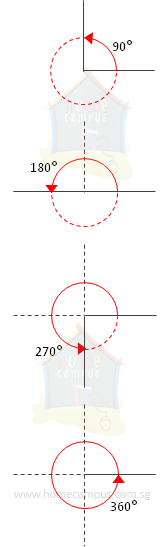#### 2. Clockwise and Anti-clockwise Directions

The direction in which the hands of a clock move is known as the clockwise direction.

The direction of movement opposite to the hands of a clock is known as the anti-clockwise or counter-clockwise direction.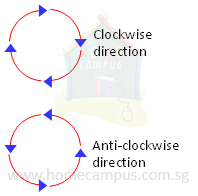#### 3. 8-Point Compass

The 8-point compass shows directions.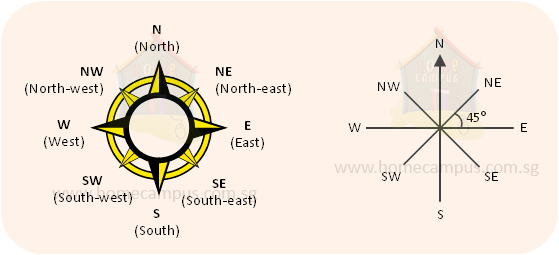#### 4. I am standing at a major crossroads as shown below in the image below. Answer the questions that follow.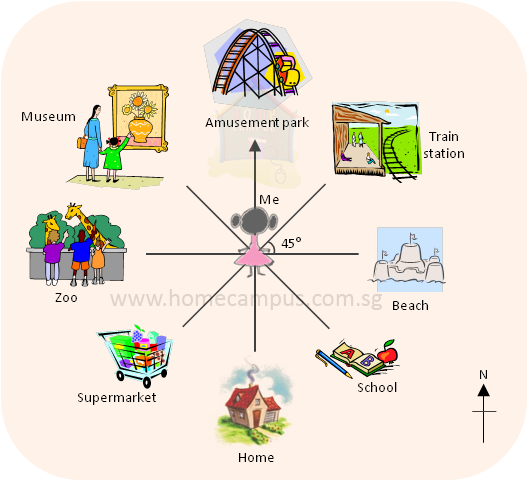a)   I am facing north. Which place is to my east?
The arrow points to the north (N) of the compass.
b)   I am looking south. If I turn 45° in the clockwise direction, what will I be facing?

c)   I am facing the zoo. How many degrees in the anti-clockwise direction must I turn to face the school?

d)   I am facing south-west. Which place will I be facing if I turn 180°?

e)   After a three-quarter turn in the clockwise direction I end up facing the supermarket. Which direction was I facing at the start?

#### 5. Fill in the blanks based on the image below.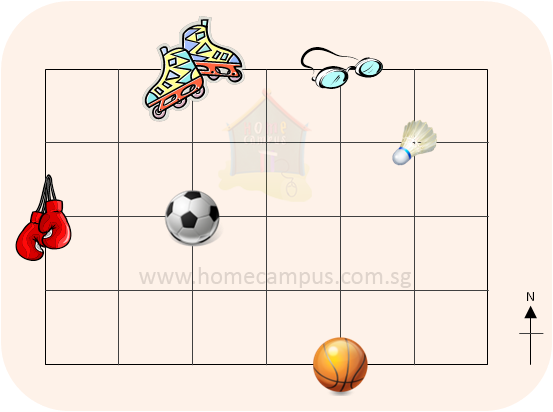a)   The ___________ is north-west of the basketball.

b)   The ___________ is south-east of the soccer ball.

c)   The ___________ are north-east of the soccer ball.